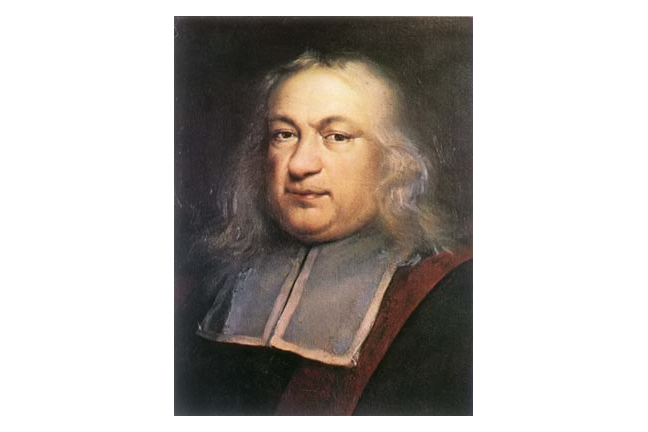## Want to keep learning?

This content is taken from the UNSW Sydney's online course, Maths for Humans: Linear, Quadratic & Inverse Relations. Join the course to learn more.
4.4

## UNSW SydneyPierre de Fermat

# Fermat and Diophantine equations

We have seen that linear equations like $$\normalsize{y=3x-4}$$ correspond to lines, and second degree equations like $$\normalsize{x^2-4xy+6y^2-x=5}$$ correspond to conic sections. In the 17th century, mathematicians asked: what do third degree equations look like? These equations arose with Fermat’s work on a famous problem from number theory and were also studied by Descartes and Newton.

In this step, we will

• learn about squares, cubes, and Fermat’s last theorem

• get some experience for what general cubic curves look like

• see that the special case of a cubic function is quite a lot simpler.

## Squares and cubes

Squares are natural numbers of the form $$\normalsize n^2$$ where $$\normalsize n$$ is an integer. They are

$\Large{1,4,9,16,25,36,49,64,81,100,121,144,169,196,225,256,289,...}.$

Recall that a Pythagorean triple occurs when two squares sum to a third square, such as the famous relation

$\Large{3^2+4^2=5^2} \quad \text {or} \quad \Large{9+16=25}.$

But there are many other examples, such as $$\normalsize{25+144=169}$$ or $$\normalsize{64+225=289}$$. In fact there are an unbounded number of Pythagorean triples — a beautiful fact that was already known to Euclid around 300 BC, and probably to the Babylonians 1500 years earlier!

Q1 (E): What are some other Pythagorean triples that you can find just in the list of squares we have given up to $$\normalsize 289$$?

Cubes are the natural numbers of the form $$\normalsize n^3$$ where $$\normalsize n$$ is an integer. They are

$\Large{1,8,27,64,125,216,343,512,729,1000, 1331,...}$

They grow much more quickly than the squares. A natural question is: can we find a “Pythagorean triple” of cubes where two of them add up to the third?

We can restate this famous question in the form: can we find solutions to the equation

$\Large{l^3+m^3=n^3}$

with natural numbers $$\normalsize{l,m,n>0}$$?

## Sums of cubes, and Fermat’s last theorem

In fact there are no such triples of numbers! This was stated by Fermat (1601 - 1665) as a challenge to his fellow mathematicians, and proved by Leonhard Euler in 1770. This kind of polynomial equation, where we are looking for natural number solutions, is called a Diophantine equation, after the mathematician Diophantus of Alexandria who lived in the fourth century, roughly 310 to 390 AD. It was a favourite topic for Fermat.

Remarkably though, we can find four cubes that have the sum property:

$\Large{3^3+4^3+5^3=6^3}.$

Fermat’s question extends to a much more ambitious claim: that in fact for any natural number $$\normalsize{n}$$ strictly greater than $$\normalsize{2}$$, there are no natural number solutions to the equation

$\Large{x^n+y^n=z^n}.$

So the quadratic situation when $$\normalsize{n=2}$$ is very different from all higher cases. This problem — called Fermat’s last theorem because Fermat famously claimed he had a proof but did not write it down — was one of the most famous unsolved problems in mathematics until 1994, when it was settled by Andrew Wiles and Richard Taylor. Perhaps ironically, cubic curves played a big role in this work.

If you are interested in this chapter of history, check out Norman’s YouTube video on the 17th century revival of number theory.

## Cubic curves and number theory

Cubic curves have some remarkable features, with deep and somewhat mysterious number theoretical properties. One of the first studied was the Fermat curve $$\normalsize{x^3+y^3=1}$$, which is a cubic analog of the unit circle $$\normalsize{x^2+y^2=1}$$, although it looks quite different.

Q2 (E): Find some points on the Fermat curve $$\normalsize{x^3+y^3=1}$$.

This curve is intimately connected with Fermat’s question about sums of cubes.

Q3 (C): What is the connection between Fermat’s number theory problem and the cubic curve $$\normalsize{x^3+y^3=1}$$?## Descartes and Newton

Fermat was not the only 17th century mathematician who became interested in cubic curves. Descartes studied what is now called the Folium of Descartes, the curve with equation

$\Large{y^3+x^3+3xy=0}.$Isaac Newton tried to organise cubics like the ancient Greek classification of conics into ellipses, parabolas and hyperbolas. He found 72 kinds of cubic curves, and he actually left out a few which were found some years later by other researchers.

Here you can have a look at Newton’s work: although it helps if you can read Latin, which was the common scientific language of the 17th century!

Newton’s list of cubics

## Some examples of cubic curves

Let’s have a look at some cubic curves. Here is the graph of

$\Large{y^2=x^3}$which is rather remarkably different from a conic — for example it has a pointy part where two different curves meet together: this is called a cusp. But if we change the equation somewhat, we get quite different possibilities:

$\Large{y^2=x^3-x}$And here is a more random cubic

$\Large{x^3-x^2y+y^2-4x=5}$where things appear even stranger. If you have GeoGebra or Desmos, you might like to play around with inputting some random cubic polynomials, and seeing what the graphs look like.

## Cubic functions are much simpler!

However once we adopt a function point of view, and require our curve to have the form $$\normalsize{y=p(x)}$$ for a cubic polynomial $$\normalsize{p(x)}$$, the possibilities become very much simpler. The simplest such cubic function is

$\Large{y=x^3}.$Here is a more general cubic

$\Large{y=x^3-x^2-2x+3}.$Notice that it exhibits an up, down, then up shape as we view it left to right. If the sign of the leading coefficent is changed, then we would see down, up, then down instead. The pure power $$\normalsize{y=x^3}$$ is something of an exception – its graph just goes up!

A2. Quickly plugging the values into the equation $$\normalsize{y^3+x^3=1}$$ shows $$\normalsize [1,0]$$ and $$\normalsize [1,0]$$ lie on the curve. In fact these are the only rational points on the curve!
A3. If $$\normalsize l,m,n$$ are natural numbers greater than zero satisfing $$\normalsize{l^3+m^3=n^3}$$, then
$\Large{(\frac{l}{n})^3+(\frac{m}{n})^3=1}$
which means that $$\normalsize{x=l/n}$$ and $$\normalsize{y=m/n}$$ are rational numbers such that the point $$\normalsize{[x,y]}$$ lies on the curve $$\normalsize{x^3+y^3=1}$$. So this gives a connection between natural number triples satisfying the Diophantine equation $$\normalsize{l^3+m^3=n^3}$$ and rational points on the cubic Fermat curve.